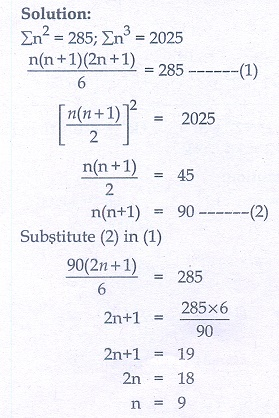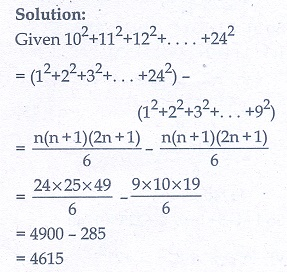Home | | Maths 10th Std | Exercise 2.9: Special Series

# Exercise 2.9: Special Series

(i) Sum of first ‘n’ natural numbers (ii) Sum of first ‘n’ odd natural numbers. (iii) Sum of squares of first ‘n’ natural numbers. (iv) Sum of cubes of first ‘n’ natural numbers. (Maths Book back answers and solution for Exercise questions)

## Exercise 2.9

1. Find the sum of the following series

(i) 1 + 2 + 3 + …  + 60

(ii) 3 + 6 + 9 + …  + 96

(iii) 51 + 52 + 53 + …  + 92

(iv) 1 + 4 + 9 + 16 + …  + 225

(v) 62 + 72 + 8 2 + …  + 212

(vi) 10 3 + 113 + 12 3 + …  + 203

(ivi) 1 + 3 + 5 + …  + 712. If 1 + 2 + 3 + …  + k = 325 , then find 13 + 23 + 33 + …  + k 3 .3. If 13 + 23 + 33 + …  + k 3 =44100 then find 1 + 2 + 3 + …  + k .4. How many terms of the series 13 + 23 + 33 + …  should be taken to get the sum 14400?5. The sum of the squares of the first n natural numbers is 285, while the sum of their cubes is 2025. Find the value of n.6. Rekha has 15 square colour papers of sizes 10 cm, 11 cm, 12 cm,…, 24 cm. How much area can be decorated with these colour papers?7. Find the sum of the series (23 − 1) + (43 − 33 ) + (63 − 153 ) + ….  to

(i) n terms (ii) 8 terms1. (i) 1830 (ii) 1584 (iii) 3003

(iv) 1240 (v) 3256 (vi) 42075 (vii) 1296

2. 105625

3. 210

4. 15

5. 9

6. 4615 cm2

7.(i) 4n2 + 3n2 (ii) 2240

Tags : Problem Questions with Answer, Solution | Mathematics , 10th Mathematics : UNIT 2 : Numbers and Sequences
Study Material, Lecturing Notes, Assignment, Reference, Wiki description explanation, brief detail
10th Mathematics : UNIT 2 : Numbers and Sequences : Exercise 2.9: Special Series | Problem Questions with Answer, Solution | Mathematics